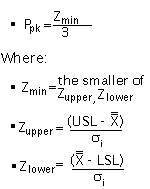## Cpk or Ppk: Which should you use?Your customer has asked you to report the Cpk of the product you are sending. You know that to compute the Cpk, you need to have the product specifications, and that you need to have the mean and sigma. As you gather the information, someone asks, “Which sigma do they want?”

You know that Cpk is calculated by dividing by 3 sigma. But which sigma should you use, estimated or calculated? Which is correct? Which would you report? Naturally, most of us would use the sigma that makes the Cpk look the best. But the sigma that makes the Cpk look best may not accurately reflect what you or your customer need to know about the process.

Confusion over calculating Cpk by two different methods is one reason that a new index, Ppk, was developed. Ppk uses the calculated sigma from the individual data.Sigma of the individuals:Given that Ppk uses the calculated sigma, it is no longer necessary to use the calculated sigma in Cpk. The only acceptable formula for Cpk uses the estimated sigma.Estimated sigma:Given that Ppk uses the calculated sigma, it is no longer necessary to use the calculated sigma in Cpk. The only acceptable formula for Cpk uses the estimated sigma.

In 1991, the ASQC/AIAG Task Force published the “Fundamental Statistical Process Control” reference manual, which shows the calculations for Cpk as well as Ppk. These should be used to eliminate confusion about calculating Cpk.

So which value is best to report, Cpk or Ppk? Although they show similar information, they have slightly different uses.

Estimated sigma and the related capability indices (Cp, Cpk, and Cr) are used to measure the potential capability of a system to meet customer needs. Use it when you want to analyze a system’s aptitude to perform.

Actual or calculated sigma (sigma of the individuals) and the related indices (Pp, Ppk, and Pr) are used to measure the performance of a system to meet customer needs. Use it when you want to measure a system’s actual process performance.

Once you determine which capability index you will use, it can easily be calculated using software such as SQCpack.